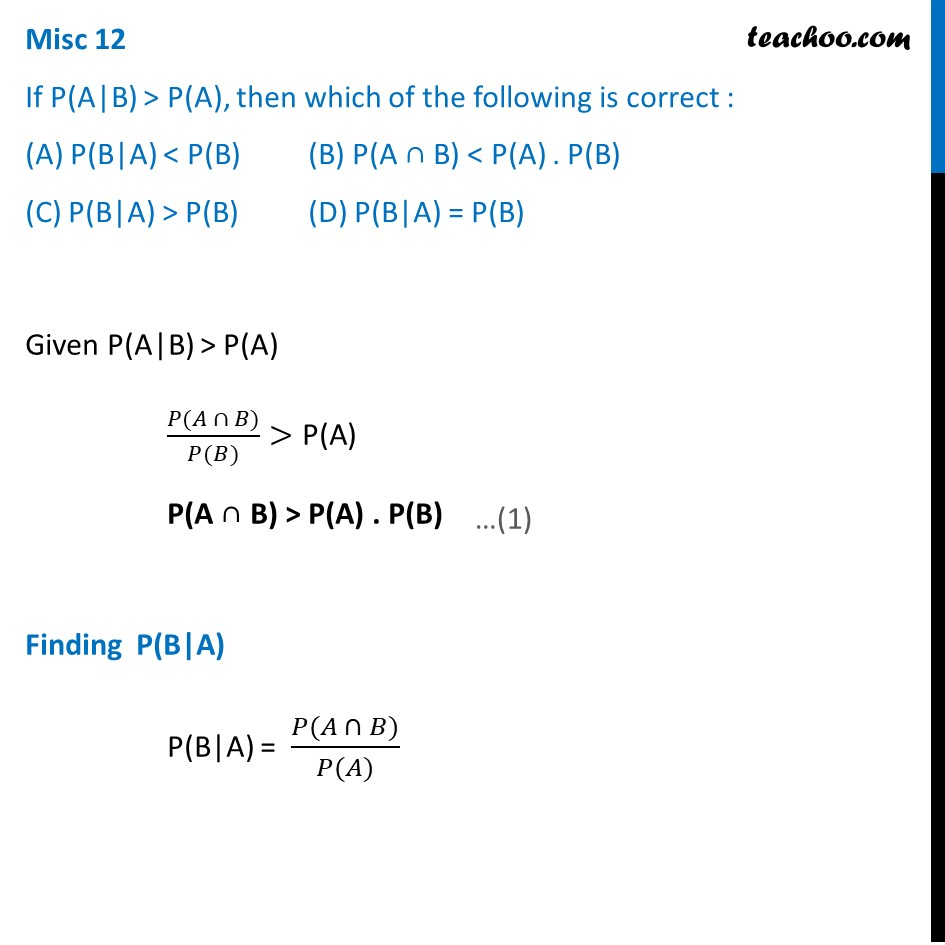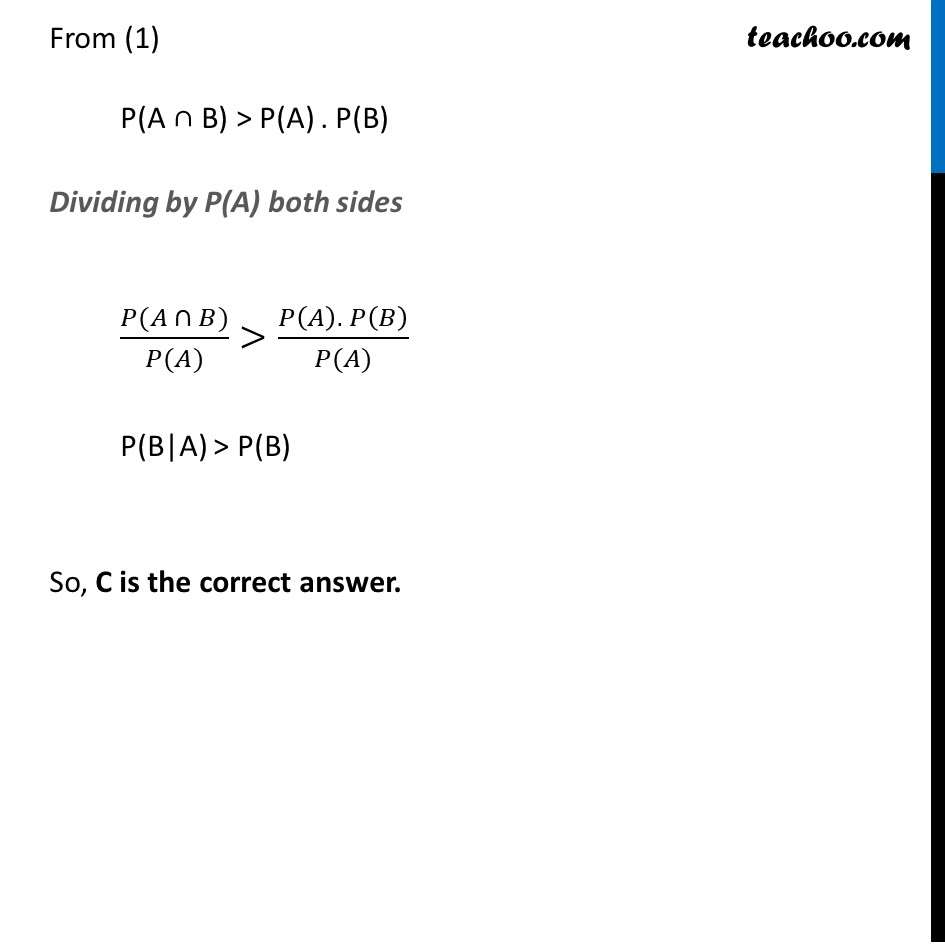Miscellaneous

Chapter 13 Class 12 Probability
Serial order wiseLearn in your speed, with individual attention - Teachoo Maths 1-on-1 Class

### Transcript

Misc 12 If P(A|B) > P(A), then which of the following is correct : (A) P(B|A) < P(B) (B) P(A ∩ B) < P(A) . P(B) (C) P(B|A) > P(B) (D) P(B|A) = P(B) Given P(A|B) > P(A) (𝑃(𝐴 ∩ 𝐵))/(𝑃(𝐵))>"P(A") P(A ∩ B) > P(A) . P(B) Finding P(B|A) P(B|A) = (𝑃(𝐴 ∩ 𝐵))/(𝑃(𝐴)) From (1) P(A ∩ B) > P(A) . P(B) Dividing by P(A) both sides (𝑃(𝐴 ∩ 𝐵))/(𝑃(𝐴))>(𝑃(𝐴). 𝑃(𝐵))/(𝑃(𝐴)) P(B|A) > P(B) So, C is the correct answer.